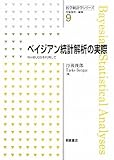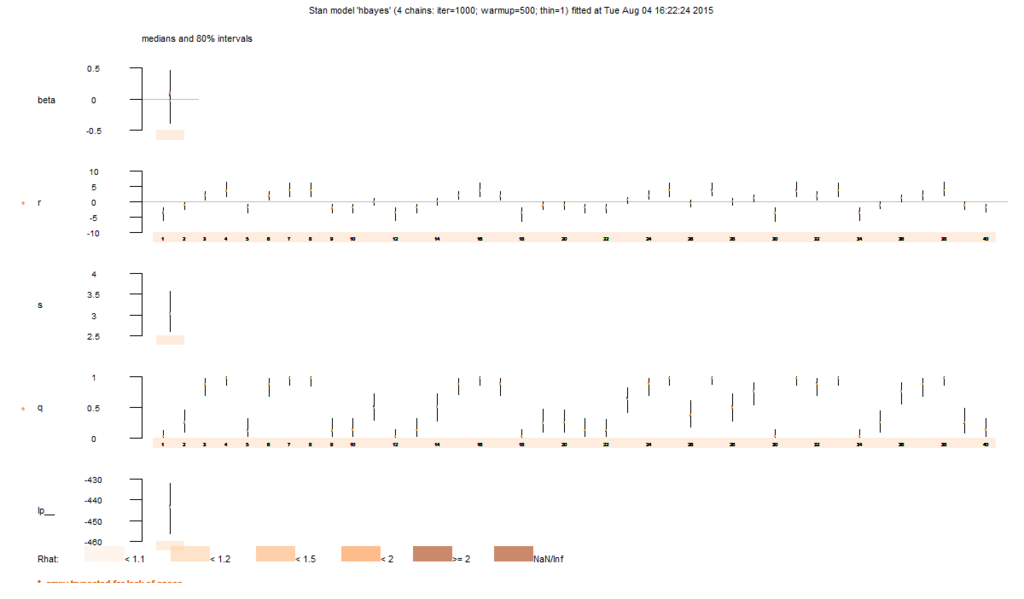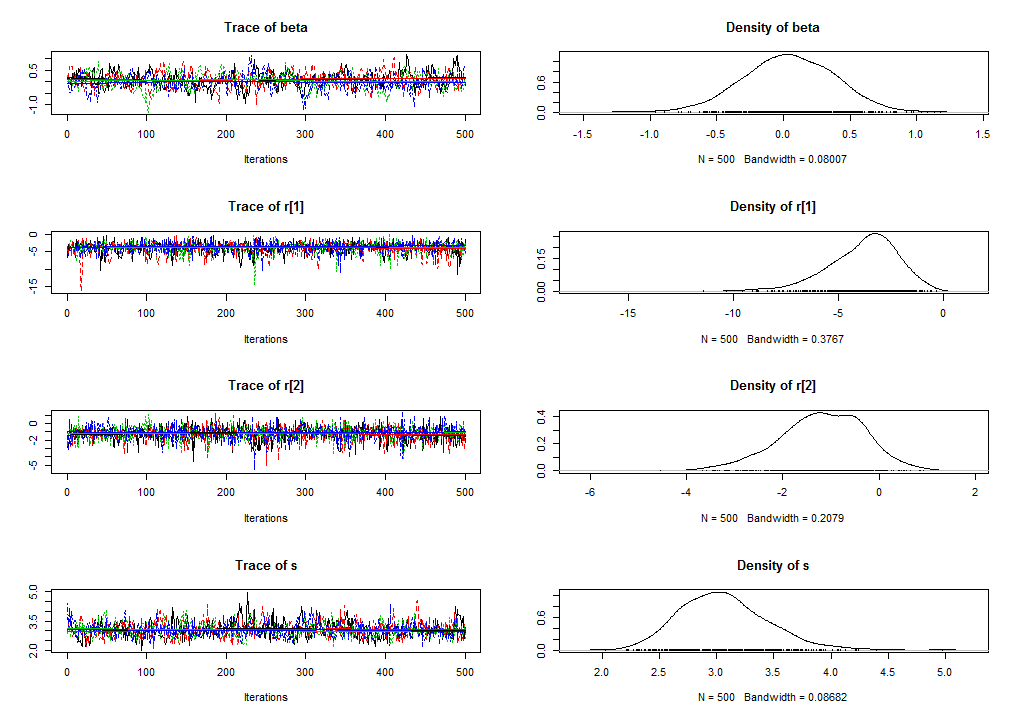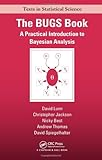# Bayesian modeling with R and Stan (3): Simple hierarchical Bayesian model

In 2 previous posts, you learned what Bayesian modeling and Stan are and how to install them. Now you are ready to try it on some very Bayesian problems - as many people love - such as hierarchical Bayesian model.

### Definition of hierarchical Bayesian models

Prior to tackling with a practical example, let's overview what and how hierarchical Bayesian model is. A famous book on Bayesian modeling with MCMC, written by Toshiro Tango and Taeko Becque and published in Japan, describes as below*1.ベイジアン統計解析の実際 (医学統計学シリーズ)

In a fixed-effects model of frequentist, each result is assumed to have a common average.On the other hand, in a random-effects model, each result is assumed to have a distinct averageand it is distributed around a global average.Bayesian hierarchical models assume prior probability for parametersof a probability distribution ofin a random-effects model, such asIt is said that such models have a hierarchical structure with two levels, that is,

• 1st level: a probability distribution is assumed for• 2nd level: one more probability distribution is assumed for parameters of the 1st levelThis is a textbook definition of hierarchical models, but I think it can be understood more intuitively; in hierarchical Bayesian models, often the models have to handle some excessive fluctuations as nonlinear effects more than expected in usual frequentist's models. Priors used in such models can be seen as an "absorber" that can absorb various kinds of fluctuations distributed around true parameters.

### A simple practice of an univariate logistic regression model with random effects

In this post, I follow an example from another famous textbook written by Takuya Kubo.

This example assumes an experiment in which we take 8 seeds from each of a certain 100 plants with a various number of leaves (unknown), thus its dataset contains 1) ID of each plant and 2) the number of survived seeds in 100 rows. From the viewpoint of hierarchical Bayesian modeling, unknown number of leaves of each plant can be random effects*2. You can get it with R as below.

> d <- read.csv(url("http://hosho.ees.hokudai.ac.jp/~kubo/stat/iwanamibook/fig/hbm/data7a.csv"))
id y
1  1 0
2  2 2
3  3 7
4  4 8
5  5 1
6  6 7


Next, prepare a Stan script. Please write a code like below and save it as "hbayes.stan".

data {
int<lower=0> N; // sample size
int<lower=0> y[N]; // the number of survived seeds for each set of 8 seeds (dependent variable)
}
parameters {
real beta; // a coefficient of logistic regression model common across all individual seeds
real r[N]; // individual bias (random effects), perhaps given from the number of leaves of each plant
real<lower=0> s; // individual deviation
}
transformed parameters {
real q[N];
for (i in 1:N)
q[i] <- inv_logit(beta+r[i]); // inverse logit of survival probability
}
model {
for (i in 1:N)
y[i] ~ binomial(8,q[i]); // logistic regression using binomial sampling
beta~normal(0,1.0e+2); // uninformative prior of coefficient
for (i in 1:N)
r[i]~normal(0,s); // hierarchical prior of individual bias (random effects)
s~uniform(0,1.0e+4); // uninformative prior for r[i]
}


Please see some important points in this Stan script. The transformed parameters block contains a transform of dependent variable from raw data to an inverse logit, but with individual biases (random effects). The model block contains some sampling statements in addition to an usual sampling procedure of logistic regression.

The order of statements in the model block may affect its result, even some changes cause compilation error. In principle an order of sampling statements would affect a structure of computation in the model block.

OK, now we can sample each parameter with Stan. Just run as below.

> dat<-list(N=nrow(d),y=d\$y)
> d.fit<-stan(file='hbayes.stan',data=dat,iter=1000,chains=4)
TRANSLATING MODEL 'hbayes' FROM Stan CODE TO C++ CODE NOW.
COMPILING THE C++ CODE FOR MODEL 'hbayes' NOW.
cygwin warning:
MS-DOS style path detected: C:/PROGRA~1/R/R-30~1.2/etc/i386/Makeconf

# (a lot of warnings) #

SAMPLING FOR MODEL 'hbayes' NOW (CHAIN 1).

# ... #

SAMPLING FOR MODEL 'hbayes' NOW (CHAIN 4).

Iteration:   1 / 1000 [  0%]  (Warmup)

Informational Message: The current Metropolis proposal is about to be rejected because of the following issue:
Error in function stan::prob::normal_log(N4stan5agrad3varE): Scale parameter is 0:0, but must be > 0!
If this warning occurs sporadically, such as for highly constrained variable types like covariance matrices, then the sampler is fine,
but if this warning occurs often then your model may be either severely ill-conditioned or misspecified.

Iteration: 100 / 1000 [ 10%]  (Warmup)
Iteration: 200 / 1000 [ 20%]  (Warmup)
Iteration: 300 / 1000 [ 30%]  (Warmup)
Iteration: 400 / 1000 [ 40%]  (Warmup)
Iteration: 500 / 1000 [ 50%]  (Warmup)
Iteration: 501 / 1000 [ 50%]  (Sampling)
Iteration: 600 / 1000 [ 60%]  (Sampling)
Iteration: 700 / 1000 [ 70%]  (Sampling)
Iteration: 800 / 1000 [ 80%]  (Sampling)
Iteration: 900 / 1000 [ 90%]  (Sampling)
Iteration: 1000 / 1000 [100%]  (Sampling)
#  Elapsed Time: 2.49 seconds (Warm-up)
#                1.358 seconds (Sampling)
#                3.848 seconds (Total)


Successfully we now get a result. Let's see its summary.

> d.fit
Inference for Stan model: hbayes.
4 chains, each with iter=1000; warmup=500; thin=1;
post-warmup draws per chain=500, total post-warmup draws=2000.

mean se_mean   sd    2.5%     25%     50%     75%   97.5% n_eff Rhat
beta      0.04    0.02 0.35   -0.66   -0.18    0.04    0.28    0.70   312 1.02
r     -3.84    0.04 1.77   -7.94   -4.82   -3.58   -2.64   -1.05  2000 1.00
r     -1.19    0.02 0.90   -3.13   -1.75   -1.16   -0.55    0.42  2000 1.00
r      2.01    0.02 1.11    0.13    1.25    1.89    2.67    4.56  2000 1.00

# ... #

r    -0.04    0.02 0.82   -1.59   -0.58   -0.07    0.49    1.58  2000 1.00
r     2.01    0.02 1.08    0.17    1.27    1.89    2.65    4.45  2000 1.00
r   -3.84    0.04 1.75   -7.96   -4.85   -3.59   -2.61   -1.12  2000 1.00
s         3.06    0.02 0.38    2.41    2.79    3.03    3.29    3.91   525 1.00
q      0.05    0.00 0.07    0.00    0.01    0.03    0.07    0.25  2000 1.00
q      0.27    0.00 0.14    0.05    0.16    0.25    0.36    0.57  2000 1.00
q      0.85    0.00 0.11    0.56    0.79    0.87    0.94    0.99  2000 1.00

# ... #

q     0.50    0.00 0.17    0.19    0.38    0.50    0.62    0.81  2000 1.00
q     0.85    0.00 0.11    0.58    0.80    0.87    0.93    0.99  2000 1.00
q    0.05    0.00 0.07    0.00    0.01    0.03    0.07    0.24  2000 1.00
lp__   -444.05    0.51 9.78 -465.01 -450.24 -443.62 -437.20 -426.53   372 1.00

Samples were drawn using NUTS(diag_e) at Tue Aug 04 16:22:24 2015.
For each parameter, n_eff is a crude measure of effective sample size,
and Rhat is the potential scale reduction factor on split chains (at
convergence, Rhat=1).
> plot(d.fit)You can see how random effects r[i] fluctuates across individual plants. Even if you don't know how much the number of leaves of each plant varies, you'll guess it can be caused by some obvious individual biases, including the number of leaves.

Finally, although almost all Rhat values show good convergence, let's check its convergence.

> d.fit.coda<-mcmc.list(lapply(1:ncol(d.fit),function(x) mcmc(as.array(d.fit)[,x,])))
> plot(d.fit.coda[,c(1:3,102)])These estimated values (giving a mode of the posterior distribution of each parameter) are entirely the same as written in Kubo's book. Yeah, it's perfect. :)

### Conclusions

We learned some points in this post as below:

• Hierarchical Bayesian models assume various kinds of individual biases (random effects)
• It can be regarded as an "absorber" that can absorb fluctuations given by such random effects
• Stan can easily handle it, but be careful for writing the model block

In practical modeling, how to set hierarchical structures and how to give (un)informative priors would determine whether its model fits well or not. It requires a lot of trials and errors for everybody, but you can get some tips from a textbook below.The BUGS Book: A Practical Introduction to Bayesian Analysis (Chapman & Hall/CRC Texts in Statistical Science)

"The BUGS Book" is incredibly useful but it only assumes WinBUGS, not Stan. Please be careful for interpreting BUGS codes into Stan codes.

*1:Its original text is of course in Japanese, so this is just my own interpretation

*2:In binomial models, it's called "overdispersion"